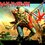# Introduction to Perfect Numbers

The history of the theory of numbers abounds with famous conjectures and open questions.Some of these have been answered some have not.This set focuses on some intriguing discussions about perfect numbers.

$\textbf{Definition:}$

A positive integer $n$ is called perfect if $n$ is equal to the sum of all of it's positive divisor's excluding itself.

$\textbf{Example:}$ 6 is a perfect number since it's divisors $3,2,1$ add up to $6$,that is, $3+2+1 = 6$.In other words $\sigma(6) = 1+2+3+6 = 12 = 2*6$

In number-theoretic notation,$\sigma(n)$ is used to denote the sum of positive divisors of a number ,hence in case of perfect numbers $\sigma(n) = 2n$

The first instances of the usage of perfect numbers dates back to Pythagoras and his school who tended to associate mystical properties with these perfect numbers. For many centuries Philosophers were more concerned about the mystical and religious significance of perfect numbers rather than their mathematical properties.Saint Augustine explains that although God could have created the world in an instant he preferred 6 days to symbolize perfection. The significance of this statement is left for the reader to decide.Note by Eddie The Head
7 years, 2 months ago

This discussion board is a place to discuss our Daily Challenges and the math and science related to those challenges. Explanations are more than just a solution — they should explain the steps and thinking strategies that you used to obtain the solution. Comments should further the discussion of math and science.

When posting on Brilliant:

• Use the emojis to react to an explanation, whether you're congratulating a job well done , or just really confused .
• Ask specific questions about the challenge or the steps in somebody's explanation. Well-posed questions can add a lot to the discussion, but posting "I don't understand!" doesn't help anyone.
• Try to contribute something new to the discussion, whether it is an extension, generalization or other idea related to the challenge.
• Stay on topic — we're all here to learn more about math and science, not to hear about your favorite get-rich-quick scheme or current world events.

MarkdownAppears as
*italics* or _italics_ italics
**bold** or __bold__ bold
- bulleted- list
• bulleted
• list
1. numbered2. list
1. numbered
2. list
Note: you must add a full line of space before and after lists for them to show up correctly
paragraph 1paragraph 2

paragraph 1

paragraph 2

[example link](https://brilliant.org)example link
> This is a quote
This is a quote
    # I indented these lines
# 4 spaces, and now they show
# up as a code block.

print "hello world"
# I indented these lines
# 4 spaces, and now they show
# up as a code block.

print "hello world"
MathAppears as
Remember to wrap math in $$ ... $$ or $ ... $ to ensure proper formatting.
2 \times 3 $2 \times 3$
2^{34} $2^{34}$
a_{i-1} $a_{i-1}$
\frac{2}{3} $\frac{2}{3}$
\sqrt{2} $\sqrt{2}$
\sum_{i=1}^3 $\sum_{i=1}^3$
\sin \theta $\sin \theta$
\boxed{123} $\boxed{123}$

## Comments

Sort by:

Top Newest

Wow!!! Thanks for giving this information.

- 7 years, 1 month ago

Log in to reply

Thanks!!I recommend you to solve the entire set on perfect numbers!! click here

- 7 years, 1 month ago

Log in to reply

very good information............

- 7 years, 1 month ago

Log in to reply

×

Problem Loading...

Note Loading...

Set Loading...